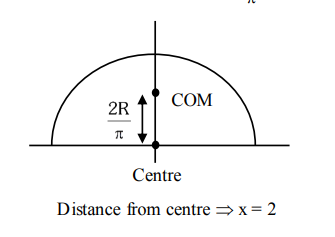# The position of the centre of mass of a uniform semi-circular wire of radius`
Question:

The position of the centre of mass of a uniform semi-circular wire of radius ' $R$ ' placed in $\mathrm{x}-\mathrm{y}$ plane with its centre at the origin and the line joining its

ends as $x$-axis is given by $\left(0, \frac{x R}{\pi}\right)$.

Then, the value of $|x|$ is________________

Solution:

COM of semi-circular ring is at $\frac{2 R}{\pi}$